SB SDK 1.11
sb_t_sample Struct Reference

Sample of an image. More...

#include <sb.h>

Collaboration diagram for sb_t_sample: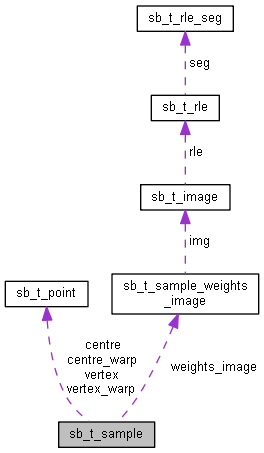[legend]

## Data Fields

char uuid 
uuid of the sample.

char image_name [SB_PAR_STRING_LEN]
Name of the image file with extension without path.

sb_t_point centre
Coordinates of the centre of the sample. More...

sb_t_point centre_warp
Coordinates of the centre in the warped image. More...

sb_t_point vertex 
Coordinates of the 4 vertices of the box of the sample. More...

sb_t_point vertex_warp 
Coordinates of the 4 vertices of the box of the sample in the warped image. More...

char model_name [SB_PAR_STRING_LEN]
Name of the model.

float scale
Scale factor. More...

sb_t_obj_type type
Sample type.

float weight
Weight of classification. More...

sb_t_sample_weights_image weights_image
Image of the weights of the sample. More...

sb_t_truth truth
Truth of the sample. More...

sb_t_sample_classify_mode classify_mode
Classification mode. More...

void * user_data
Pointer to the user data. More...

int user_data_size
Size, in bytes, of sb_t_sample::user_data buffer.

int is_near
FALSE NEGATIVE with some other sample close to it. More...

float IoU

float defect_area_percentage

## Detailed Description

Sample of an image.

It is contained both in the structure sb_t_samples and in the sb_t_res one.
In the first case it represents a sample of the ground truth, in the second an occurrence detected during the elaboration.

Some parameters are used only in one case and others may have a significance a bit different in the two cases.

Definition at line 7437 of file sb.h.

## ◆ centre

 sb_t_point sb_t_sample::centre

Coordinates of the centre of the sample.

sb_t_sample::centre_warp

Definition at line 7446 of file sb.h.

## ◆ centre_warp

 sb_t_point sb_t_sample::centre_warp

Coordinates of the centre in the warped image.

If you analyze the original image, it is equal to the parameter centre.
When a pre-elaboration operation is applied on the original image to warp it, then centre and centre_warp will be different.
centre_warp represents the coordinates of the sample in the warped image.
The function sb_svl_run every time calculates the centre_warp using the lut.
The function sb_project_detection uses the centre_warp as the coordinate of the ground truth sample.
Actually centre is only used for visualization of the sample on the original image.
In the following figure an example of warping process on an image is reported.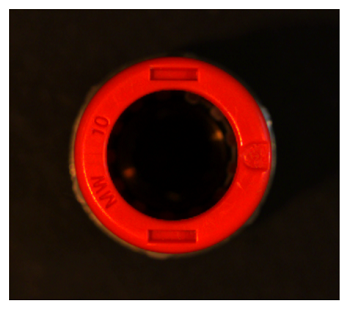Example of an object on which apply the "warping"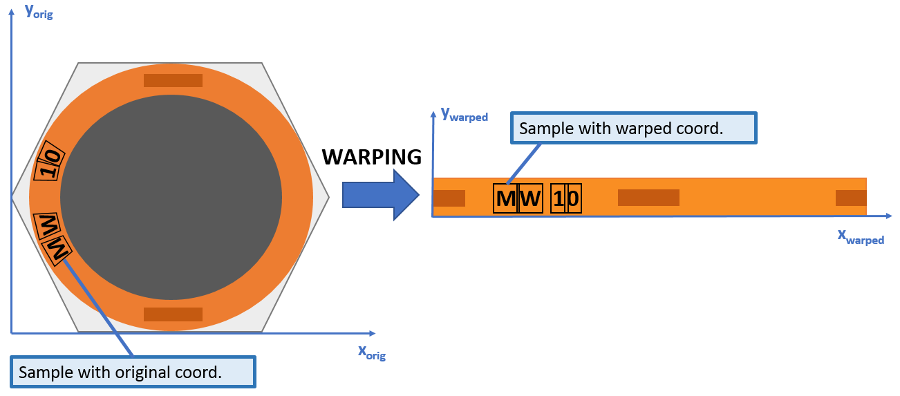Detail of the "warping" process
SVL Callbacks
Lut
sb_t_sample::vertex_warp
sb_lut_warp_point

Definition at line 7468 of file sb.h.

## ◆ classify_mode

 sb_t_sample_classify_mode sb_t_sample::classify_mode

Classification mode.

Refers to the ground truth property of a sample.
If the value is set to Required the sample is actually considered as an occurence of a model and it must be found, otherwise it will be classified as False Negative.
If the value is set to Optional means that the user is not sure about its truth value, because he does not know if it is a positive or negative instance. Sometimes in Retina it is advisable also to include in this category also all those object instances of which the user surely knows their model but they are "extreme" to classify. This is the case of objects that are partially or strongly occluded, smaller than Object Size (highly under-resoluted), blurred, etc... In the following image an example is reported.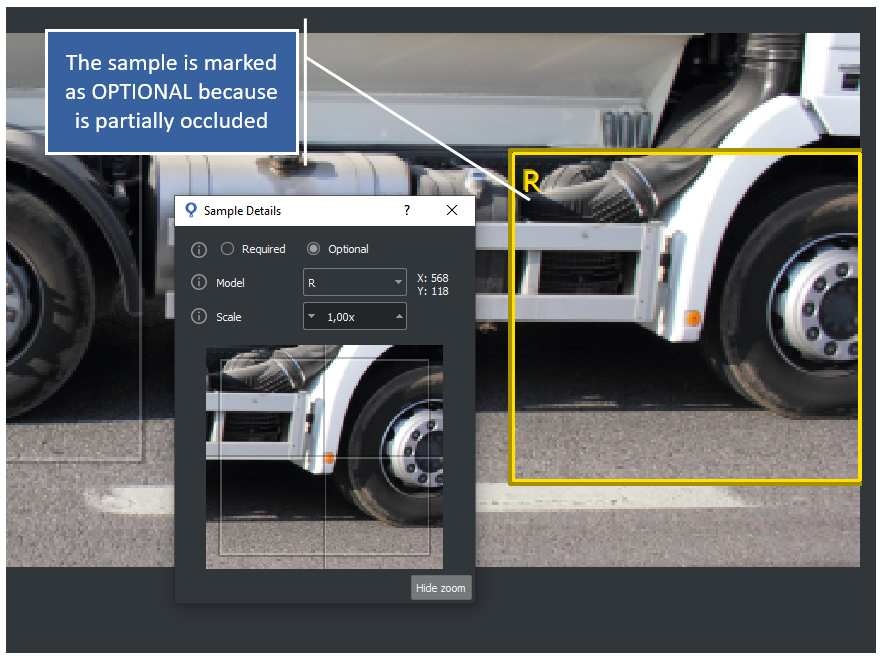Example of an OPTIONAL SAMPLE associated to a partially occluded instance

An optional sample will be classified as Optional Positive or Optional Negative depending if it is found or not. In this case, its classification has no effect on the statistics (Accuracy, Recall, Precision and Specificity).
During the SVL the Optional sample is not actually used to train the classifier, that is the region of the image is excluded by any processing, like it was not included in the ROI. However, the great advantage to collect all these sample in a category is to have a complete control over the samples and in every moment modify their Classification Mode to Required in according to a change of project specification.
In the test phase, the use of Optional has also the convenience that the user can check at any time how the current training works on such istances and sometimes being adviced about their classification from the classifier itself.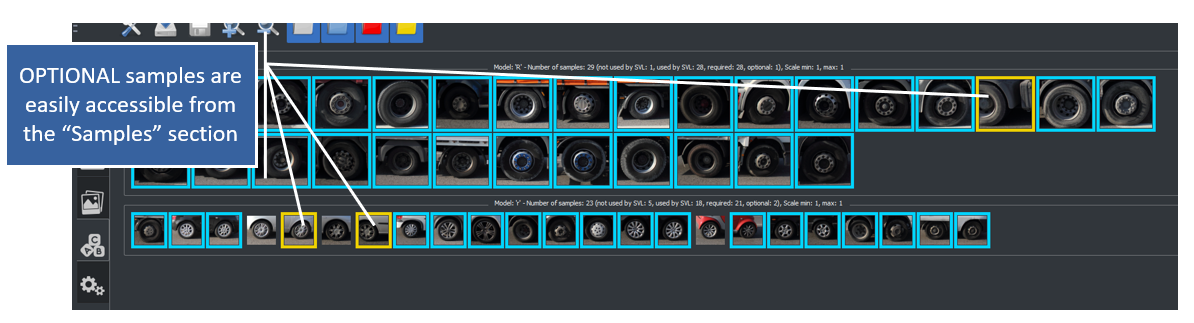OPTIONAL SAMPLES in the section "SVL samples"
sb_t_par_model.obj_size

Definition at line 7593 of file sb.h.

## ◆ defect_area_percentage

 float sb_t_sample::defect_area_percentage

Percentage of the pixels of the sample rectangle that have a weight below threshold

Definition at line 7652 of file sb.h.

## ◆ IoU

 float sb_t_sample::IoU

Percentage of overlap with the sample of ground truth.

The percentage is evaluated using Intersection over Union method.
The formula is: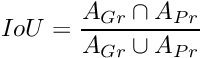where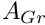is the area of the ground truth sample and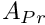is the area of the prediction.
Ihe IoU index takes into account both the overlap between the bounding box and the scale difference.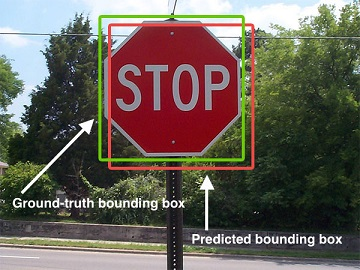Intersection over Union

It is set by the function sb_project_detection . The range of value is [0, 1.0].

• 0 means non intersection
• 1 means full overlap

The IoU parameter has been added to the sample information to help detect situations where the classifier does not find the occurrence well superimposed on the ground truth sample.
Some of the possible causes and solutions are explained in the A low IoU value . The SB GUI, at detection time, shows a warning signal on the sample if its IoU value falls below a threshold that can be set in the Settings->Advanced menu as shown in the image below.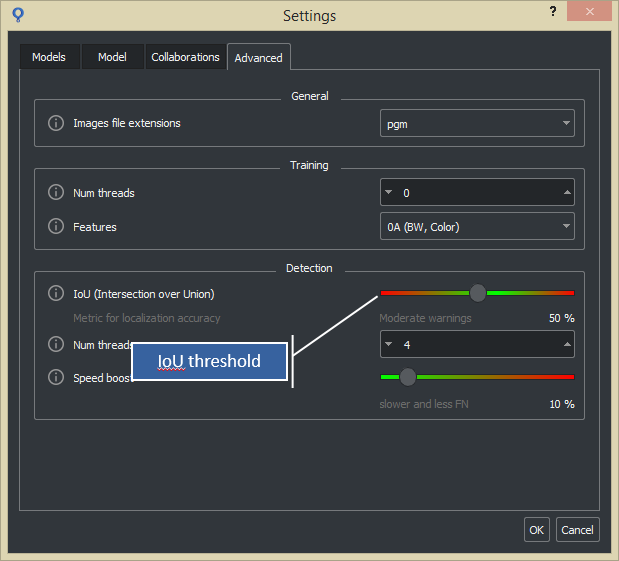IoU threshold

The following image shows the warning that is shown on the sample. A tooltip refers the user to the Settings->Advanced menu where it is possible to set the threshold and access the documentation.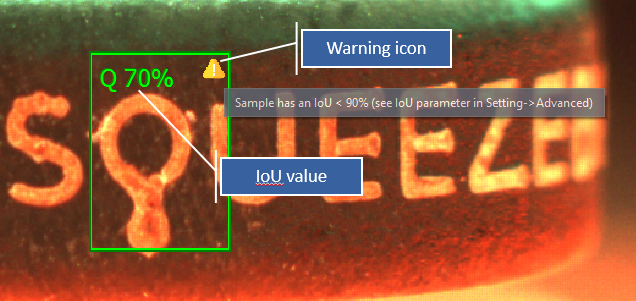IoU warning

To show the IoU value enable "Show IoU" option in the Preferences menu as shown in the image below.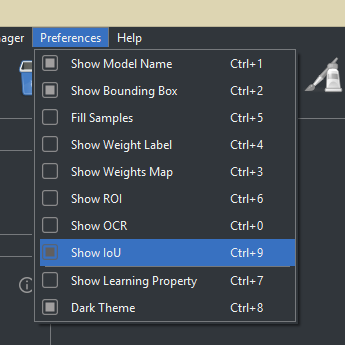A low IoU value

Definition at line 7647 of file sb.h.

## ◆ is_near

 int sb_t_sample::is_near

FALSE NEGATIVE with some other sample close to it.

In case truth is equal to SB_TRUTH_FALSE_NEGATIVE, the flag indicates if there is some sample (with truth equal to SB_TRUTH_TRUE_POSITIVE) closer than the allowed minimum distance. It is set by the function sb_project_detection .

sb_project_get_res

Definition at line 7614 of file sb.h.

## ◆ scale

 float sb_t_sample::scale

Scale factor.

The scale factor is used when the size of the model is smaller than the size of its occurrence on the image.
For Retina projects the scale factor can only be a multiple of SB_SAMPLE_SCALE_GRANULARITY.
In case the sample is returned from a Surface project, the scale can only be a multiple of SB_PAR_LEVEL_SCALE_GRANULARITY.
The range of values is between SB_SAMPLE_SCALE_MIN and SB_SAMPLE_SCALE_MAX.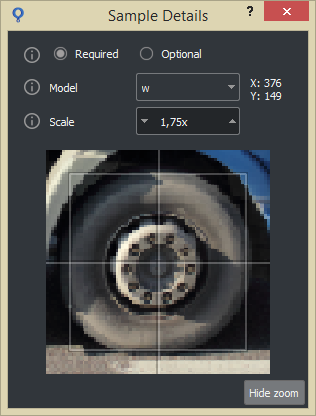Sample with scale greater than 1
sb_t_par_model::obj_size

Definition at line 7524 of file sb.h.

## ◆ truth

 sb_t_truth sb_t_sample::truth

Truth of the sample.

It is evaluated only when the sample is retrieved with the function sb_project_get_res and the function sb_project_detection was called with the samples parameter other than NULL.

Definition at line 7565 of file sb.h.

## ◆ user_data

 void* sb_t_sample::user_data

Pointer to the user data.

The buffer must be allocated with sb_malloc or sb_calloc functions.
it is mandatory to also set the parameter sb_t_sample::user_data_size otherwise the functions sb_samples_clone , sb_sample_clone wil return the SB_ERR_MALLOC error.

Definition at line 7600 of file sb.h.

## ◆ vertex

 sb_t_point sb_t_sample::vertex

Coordinates of the 4 vertices of the box of the sample.

The vertices are enumerated in the following order:

• 0=upper left
• 1=upper right
• 2=lower right
• 3=lower left

This parameter is used only if the sample is an occurrence.

sb_t_sample::vertex_warp

Definition at line 7481 of file sb.h.

## ◆ vertex_warp

 sb_t_point sb_t_sample::vertex_warp

Coordinates of the 4 vertices of the box of the sample in the warped image.

The vertices are enumerated in the following order:

• 0=upper left
• 1=upper right
• 2=lower right
• 3=lower left

If you analyze the original image, it is equal to the parameter vertex.
When a pre-elaboration operation is applied on the original image to warp it, then vertex and vertex_warp will be different.
vertex_warp represents the coordinates of the vertices of the sample in the warped image.
vertex and vertex_warp are used only to show the sample bounding box respectively on the original image and on the warped one.
Note that all the 4 vertices are needed to correctly identify the sample, because rectangular bounding boxes assumes trapezoidal shapes under warping transformations.
See images in description of sb_t_sample::centre_warp

This parameter is used only if the sample is an occurrence.

SVL Callbacks
Lut
sb_t_sample::centre_warp
sb_lut_warp_point

Definition at line 7506 of file sb.h.

## ◆ weight

 float sb_t_sample::weight

Weight of classification.

It is evaluated only when the sample is retrieved with the function sb_project_get_res.
The range of values ​​is from -1 to 1.
It can have the following value:

Definition at line 7543 of file sb.h.

## ◆ weights_image

 sb_t_sample_weights_image sb_t_sample::weights_image

Image of the weights of the sample.

It is evaluated only when the sample is retrieved with the function sb_project_get_res and the parameter details is set to 1.
The image is evaluated for the samples that are labeled as:

Definition at line 7558 of file sb.h.

The documentation for this struct was generated from the following file: